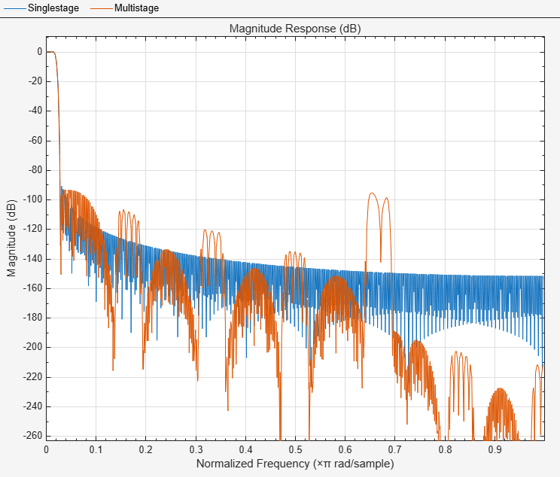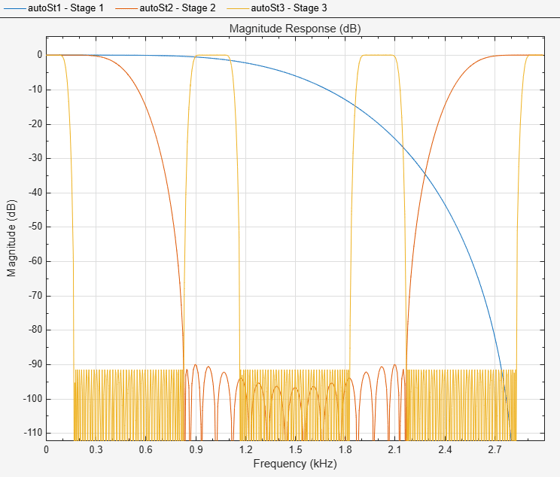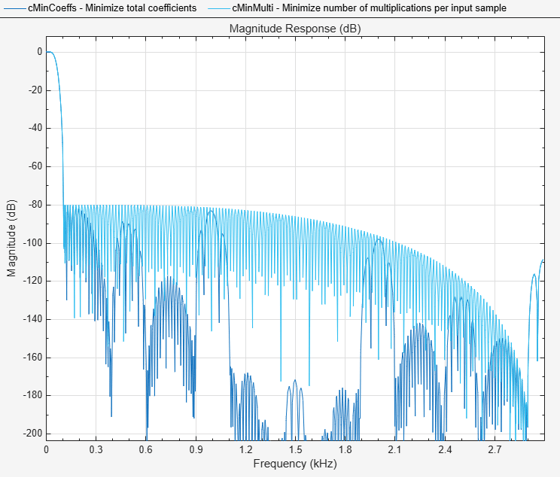designMultistageDecimator

Multistage decimator design

Description

C = designMultistageDecimator(M) designs a multistage decimator that has an overall decimation factor of M. In order for C to be multistage, M must not be a prime number. For details, see Algorithms. The design process can take a while if M has many factors.

example

C = designMultistageDecimator(M,Fs,TW) designs a multistage decimator with a sampling rate of Fs and a transition width of TW. Sampling rate in this case refers to the input sampling rate of the signal before the multistage decimator.

The multistage decimator has a cutoff frequency of Fs/(2M).

example

C = designMultistageDecimator(M,Fs,TW,Astop) specifies a minimum attenuation of Astop dB for the resulting design.

example

C = designMultistageDecimator(___,Name,Value) specifies additional design parameters using one or more name-value pair arguments.

Example: C = designMultistageDecimator(48,48000,200,80,'NumStages','auto') designs a multistage decimator with the least number of multiplications per input sample (MPIS).

Examples

collapse all

Design a single-stage decimator using the designMultirateFIR function and a multistage decimator using the designMultistageDecimator function. Determine the efficiency of the two designs using the cost function. The implementation efficiency is characterized by two cost metrics - NumCoefficients and MultiplicationsPerInputSample.

Compute the cost of implementing both designs, and determine which design is more efficient. To make a comparison, design the filters such that their transition width is the same.

Initialization

Choose a decimation factor of 48, input sample rate of $30.72×48\text{\hspace{0.17em}}\mathrm{MHz}$, one-sided bandwidth of 10 MHz, and a stopband attenution of 90 dB.

M = 48;
Fin = 30.72e6*M;
Astop = 90;
BW = 1e7;

Using the designMultirateFIR Function

Designing the decimation filter using the designMultirateFIR function yields a single-stage design. Set the half-polyphase length to a finite integer, in this case 8.

HalfPolyLength = 8;
b = designMultirateFIR(1,M,HalfPolyLength,Astop);
d = dsp.FIRDecimator(M,b)
d =
dsp.FIRDecimator with properties:

DecimationFactor: 48
NumeratorSource: 'Property'
Numerator: [0 -5.7242e-08 -1.2617e-07 -2.0736e-07 -3.0130e-07 ... ]
Structure: 'Direct form'

Show all properties

Compute the cost of implementing the decimator. The decimation filter requires 753 coefficients and 720 states. The number of multiplications per input sample and additions per input sample are 15.6875 and 15.6667, respectively.

cost(d)
ans = struct with fields:
NumCoefficients: 753
NumStates: 720
MultiplicationsPerInputSample: 15.6875

Using the designMultistageDecimator Function

Design a multistage decimator with the same filter specifications as the single-stage design. Compute the transition width using the following relationship:

Fc = Fin/(2*M);
TW = 2*(Fc-BW);

By default, the number of stages given by the NumStages argument is set to 'Auto', yielding an optimal design that tries to minimize the number of multiplications per input sample.

c = designMultistageDecimator(M,Fin,TW,Astop)
c =

Stage1: [1x1 dsp.FIRDecimator]
Stage2: [1x1 dsp.FIRDecimator]
Stage3: [1x1 dsp.FIRDecimator]
Stage4: [1x1 dsp.FIRDecimator]

Calling the info function on c shows that the filter is implemented as a cascade of four dsp.FIRDecimator objects, with decimation factors of 3, 2, 2, and 4, respectively.

Compute the cost of implementing the decimator.

cost(c)
ans = struct with fields:
NumCoefficients: 78
NumStates: 99
MultiplicationsPerInputSample: 7.2708

The NumCoefficients and the MultiplicationsPerInputSample parameters are lower for the four-stage filter designed by the designMultistageDecimator function, making it more efficient.

Compare the magnitude response of both the designs.

fvtool(b,c)
legend('Single-stage','Multistage')The magnitude response shows that the transition width of both the filters is the same, making the filters comparable. The cost function shows that implementing the multistage design is more efficient compared to implementing the single-stage design.

Using the 'design' Option in the designMultistageDecimator Function

The filter can be made even more efficient by setting the 'CostMethod' argument of the designMultistageDecimator function to 'design'. By default, this argument is set to 'estimate'.

In the 'design' mode, the function designs each stage and computes the filter order. This yields an optimal design compared to the 'estimate' mode, where the function estimates the filter order for each stage and designs the filter based on the estimate.

Note that the 'design' option can take much longer compared to the 'estimate' option.

cOptimal = designMultistageDecimator(M,Fin,TW,Astop,'CostMethod','design')
cOptimal =

Stage1: [1x1 dsp.FIRDecimator]
Stage2: [1x1 dsp.FIRDecimator]
Stage3: [1x1 dsp.FIRDecimator]
Stage4: [1x1 dsp.FIRDecimator]

cost(cOptimal)
ans = struct with fields:
NumCoefficients: 70
NumStates: 93
MultiplicationsPerInputSample: 7

Design a decimator with an overall decimation factor of 24 using the designMultistageDecimator function. Design the filter in two configurations:

• Two-stage configuration - NumStages is set to 2.

• Auto configuration - NumStages is set to 'Auto'. This configuration designs a filter with the lowest number of multiplications per input sample.

Compare the cost of implementing both the configurations.

Initialization

Choose a decimation factor of 24, input sample rate of 6 kHz, stopband attenuation of 90 dB, and a transition width of $0.03×\frac{6000}{2}$.

M = 24;
Fs = 6000;
Astop = 90;
TW = 0.03*Fs/2;

Design the Filter

Design the two filters using the designMultistageDecimator function.

cAuto = designMultistageDecimator(M,Fs,TW,Astop,'NumStages','Auto')
cAuto =

Stage1: [1x1 dsp.FIRDecimator]
Stage2: [1x1 dsp.FIRDecimator]
Stage3: [1x1 dsp.FIRDecimator]

cTwo = designMultistageDecimator(M,Fs,TW,Astop,'NumStages',2)
cTwo =

Stage1: [1x1 dsp.FIRDecimator]
Stage2: [1x1 dsp.FIRDecimator]

View the filter information using the info function. The 'Auto' configuration designs a cascade of three FIR decimators with decimation factors 2, 3, and 4, respectively. The two-stage configuration designs a cascade of two FIR decimators with decimation factors 4 and 6, respectively.

Compare the Cost

Compare the cost of implementing the two designs using the cost function.

cost(cAuto)
ans = struct with fields:
NumCoefficients: 73
NumStates: 94
MultiplicationsPerInputSample: 8.6250

cost(cTwo)
ans = struct with fields:
NumCoefficients: 100
NumStates: 118
MultiplicationsPerInputSample: 8.9583

The 'Auto' configuration decimation filter yields a three-stage design that out-performs the two-stage design on all cost metrics.

Compare the Magnitude Response

Comparing the magnitude response of the two filters, both the filters have the same transition-band behavior and follow the design specifications.

fvtool(cAuto,cTwo,'magnitude')
legend('Auto multistage','Two-stage')However, to understand where the computational savings are coming from in the three-stage design, look at the magnitude response of the three stages individually.

autoSt1 = cAuto.Stage1;
autoSt2 = cAuto.Stage2;
autoSt3 = cAuto.Stage3;
fvtool(autoSt1, autoSt2, autoSt3,'magnitude')
legend('Stage 1','Stage 2','Stage 3')The third stage provides the narrow transition width required for the overall design (0.03$×$Fs/2). However, the third stage operates at 1.5 kHz and has spectral replicas centered at that frequency and its harmonics.

The first stage removes such replicas. This first and second stages operate at a faster rate but can afford a wide transition width. The result is a decimate-by-2 first-stage filter with only 7 nonzero coefficients and a decimate-by-3 second-stage filter with only 19 nonzero coefficients. The third stage requires 47 coefficients. Overall, there are 73 nonzero coefficients for the three-stage design and 100 nonzero coefficients for the two-stage design.

The filters in the multistage design satisfy the following conditions:

• The combined response must meet or exceed the given design specifications.

• The combined decimation must equal the overall decimation required.

For an overall decimation factor of 48, there are several combinations of individual stages.

To obtain a design with the least number of total coefficients, set the 'MinTotalCoeffs' argument to true.

Astop = 80;
M = 48;
Fs = 6000;
TW = 0.03*Fs/2;
cMinCoeffs = designMultistageDecimator(M,Fs,TW,Astop,'MinTotalCoeffs',true)
cMinCoeffs =

Stage1: [1x1 dsp.FIRDecimator]
Stage2: [1x1 dsp.FIRDecimator]
Stage3: [1x1 dsp.FIRDecimator]
Stage4: [1x1 dsp.FIRDecimator]

cost(cMinCoeffs)
ans = struct with fields:
NumCoefficients: 48
NumStates: 59
MultiplicationsPerInputSample: 5.8542

To obtain the design with the least number of multiplications per input sample, set 'NumStages' to 'auto'.

cMinMulti = designMultistageDecimator(M,Fs,TW,Astop,'NumStages','auto')
cMinMulti =

Stage1: [1x1 dsp.FIRDecimator]
Stage2: [1x1 dsp.FIRDecimator]

cost(cMinMulti)
ans = struct with fields:
NumCoefficients: 158
NumStates: 150
MultiplicationsPerInputSample: 5.6875

Compare the magnitude response of both filters using fvtool. Both filters have the same transition-band behavior and a stopband attenuation that is below 80 dB.

fvtool(cMinCoeffs,cMinMulti)
legend('Minimize total coefficients','Minimize number of multiplications per input sample')Input Arguments

collapse all

Overall decimation factor, specified as a positive integer greater than one. In order for C to be multistage, M must not be a prime number. For details, see Algorithms.

Data Types: single | double | int8 | int16 | int32 | int64 | uint8 | uint16 | uint32 | uint64

Input sampling rate prior to the multistage decimator, specified as a positive real scalar. If not specified, Fs defaults to 48,000 Hz. The multistage decimator has a cutoff frequency of Fs/(2M).

Data Types: single | double | int8 | int16 | int32 | int64 | uint8 | uint16 | uint32 | uint64

Transition width, specified as a positive real scalar less than Fs/M. If not specified, TW defaults to 0.2×Fs/M. Transition width must be less than Fs/M.

Data Types: single | double | int8 | int16 | int32 | int64 | uint8 | uint16 | uint32 | uint64

Minimum stopband attenuation for the resulting design, specified as a positive real scalar.

Data Types: single | double | int8 | int16 | int32 | int64 | uint8 | uint16 | uint32 | uint64

Name-Value Arguments

Specify optional comma-separated pairs of Name,Value arguments. Name is the argument name and Value is the corresponding value. Name must appear inside quotes. You can specify several name and value pair arguments in any order as Name1,Value1,...,NameN,ValueN.

Example: C = designMultistageDecimator(48,48000,200,80,'NumStages','auto') designs a multistage decimator with the lowest number of multiplications per input sample.

Number of decimator stages, specified as a positive integer. If set to 'auto', the design algorithm determines the number of stages that result in the lowest number of multiplications per input sample. If specified as a positive integer, N, the overall decimation factor, M, must be able to factor into at least N factors, not counting 1 or M as factors.

Data Types: single | double | int8 | int16 | int32 | int64 | uint8 | uint16 | uint32 | uint64

When false, the design algorithm minimizes the number of multiplications per input sample. When true, the design algorithm minimizes the total number of coefficients.

Data Types: logical

Cost computation method, specified as either:

• 'estimate' –– The function estimates the filter order required for each stage and designs the filter based on the estimate. This method is faster than 'design', but can lead to suboptimal designs.

• 'design' –– The function designs each stage and computes the filter order. This method leads to an optimal overall design.

Data Types: char

Tolerance, specified as a positive scalar. The tolerance is used to determine the multistage configuration with the least MPIS. When multiple configurations result in the same lowest MPIS within the tolerance specified, the configuration that yields the lowest number of coefficients overall is chosen. To view the total number of coefficients and MPIS for a specific filter, use the cost function.

Data Types: single | double | int8 | int16 | int32 | int64 | uint8 | uint16 | uint32 | uint64

Output Arguments

collapse all

Designed filter, returned as a dsp.FilterCascade System object™. The filter is a cascade of the multiple stages designed by the function. The number of stages is determined by the 'NumStages' argument.

To get information about each filter stage, call the info function on the C argument.

Algorithms

The overall decimation factor is split into smaller factors with each factor being the decimation factor of the corresponding individual stage. The combined decimation of all the individual stages must equal the overall decimation. The combined response must meet or exceed the given design specifications.

The function determines the number of decimator stages through the 'NumStages' argument. The sequence of stages is determined based on the implementation cost. By default, 'NumStages' is set to 'auto', resulting in a sequence that gives the lowest number of MPIS. When multiple configurations result in the same lowest MPIS within the tolerance specified, the configuration that yields the lowest number of coefficients overall is chosen. If 'MinTotalCoeffs' is set to true, the function determines the sequence that requires the lowest number of total coefficients.

By default, the 'CostMethod' is set to 'estimate'. In this mode, the function estimates the filter order required for each stage and designs the filter based on the estimate. This method is faster than 'design', but can lead to suboptimal designs. For an optimal design, set 'CostMethod' to 'design'. In this mode, the function designs each stage and computes the filter order.

Functions

Introduced in R2018b

DSP System Toolbox DocumentationGet trial now